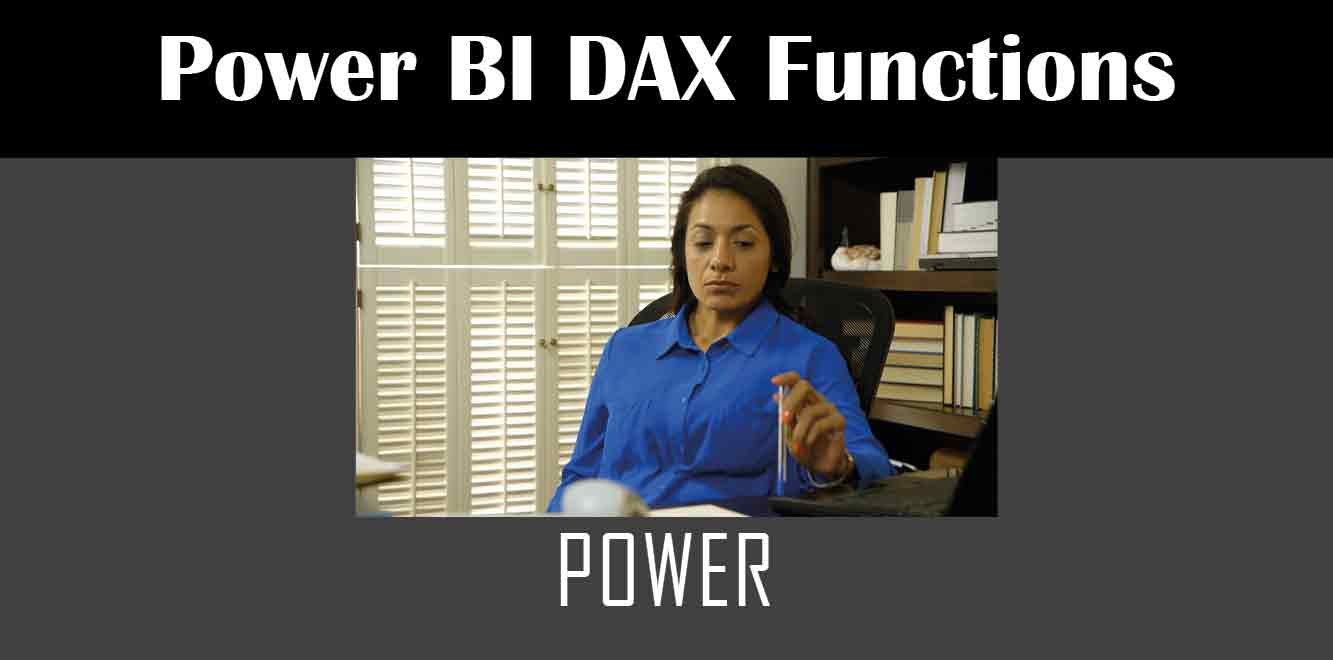# Power

## YIn Power Fx, the Power function is used to raise a number to a certain power. This can be useful in many different scenarios, such as calculating interest rates or finding the square root of a number. In this article, we will discuss how to use the Power function in Power Apps.

## Syntax of the Power Function

The Power function follows a specific syntax. The syntax of the Power function is as follows:

Power(Base, Exponent)

``` The Base argument is the number that you want to raise to a certain power. The Exponent argument is the power that you want to raise the Base to. Examples of Using the Power Function Example 1: Calculating Simple Interest Let's say you want to calculate the simple interest on a loan. The formula for calculating simple interest is as follows: ```

Simple Interest = (principal * rate * time) / 100

``` Here, the principal is the amount of money borrowed, the rate is the interest rate, and the time is the duration of the loan in years. To calculate the simple interest using the Power function, you can use the following formula: ```

Simple Interest = (principal * Power(1 + rate/100, time)) – principal

``` In this formula, the Base argument is (1 + rate/100), and the Exponent argument is the time. Example 2: Finding the Square Root of a Number If you want to find the square root of a number using the Power function, you can use the following formula: ```

Square Root = Power(Number, 0.5)

``` In this formula, the Base argument is the number that you want to find the square root of, and the Exponent argument is 0.5. Example 3: Raising a Number to a Fractional Power You can also use the Power function to raise a number to a fractional power. For example, if you want to find the cube root of a number using the Power function, you can use the following formula: ```

Cube Root = Power(Number, 1/3)

``` In this formula, the Base argument is the number that you want to find the cube root of, and the Exponent argument is 1/3. The Power function is a very useful function in Power Apps. It can be used to perform a variety of calculations, such as finding the square root of a number or raising a number to a certain power. By understanding the syntax of the Power function and its various use cases, you can harness the power of Power Fx and create powerful custom business applications. Power Apps Training Courses by G Com Solutions (0800 998 9248)1-Week Intensive Power Apps Training Course £4,975.00 Select optionsContinue Loading DonePower Apps Introduction (2 Days) £1,990.00 Select optionsContinue Loading DonePower Apps Intermediate (1 Day) £995.00 Select optionsContinue Loading DonePower Apps Advanced (2 Days) £1,990.00 Select optionsContinue Loading Done ```
``` ```
``` ```
``` ```
``` ```
``` ```